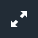# Parameter Key

To look around: click and drag somewhere other than the menu.
To zoom: use mouse scrollwheel or up/down arrow keys.
To open the menu: click the gray button on the bottom center.

### Cycle Diagram

This diagram (left part of menu) shows the 6 possible states for each motor. The transition arrows between states are labeled with the current rate of the transition (units = transitions per simulation second). Each of these rates can be adjusted by clicking and dragging the circular knob within each arrow. The states are defined as follows:

• Mt-KT = ATP bound, bound to tubulin
• Mt-KDP = ADP and Pi bound, bound to tubulin
• KDP = ADP and Pi bound, diffusing freely
• KD = ADP bound, diffusing freely
• Mt-KD = ADP bound, bound to tubulin
• Mt-K = no nucleotide, bound to tubulin

### Average Velocity

Calculated by dividing the distance travelled by the time passed since start (or restart).

### Time

How much slower the simulation is running compared to real time.

### Mean Step Size

The average distance a freely diffusing molecule moves in one step. Each step, this distance is randomly sampled from an exponential distribution with this number as the mean.